Symbol
Problem$1.$ Tentukan himpunan penyelesaian dari $\dfrac {2x+3} {x-3}\leq 31$ $2.$ Tentukan himpunan penyelesaian dari $\dfrac {2x^{2}-17x-9} {2x^{2}+7x+3}\geq 0$ $3$ Tentukan himpunan penyelesaian dari $\sqrt{3x-7} \geq x-1y$ $4.$ Penyelesaian pertidaksamaan $\sqrt{x^{2}-x-12} >\sqrt{x+3}$
Other
Search count: 111
SolutionQanda teacher - SHIVRAJ
Which question?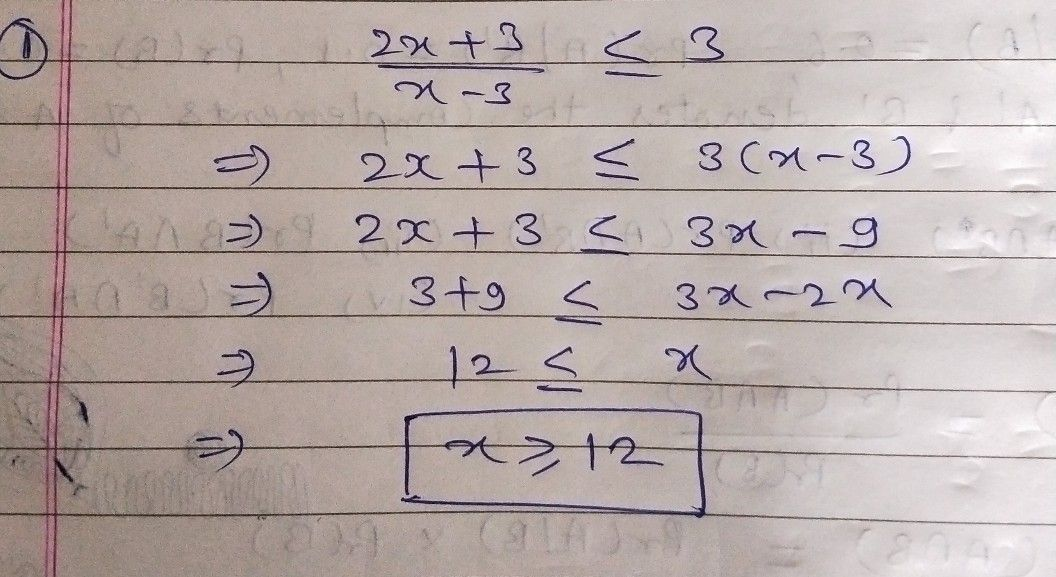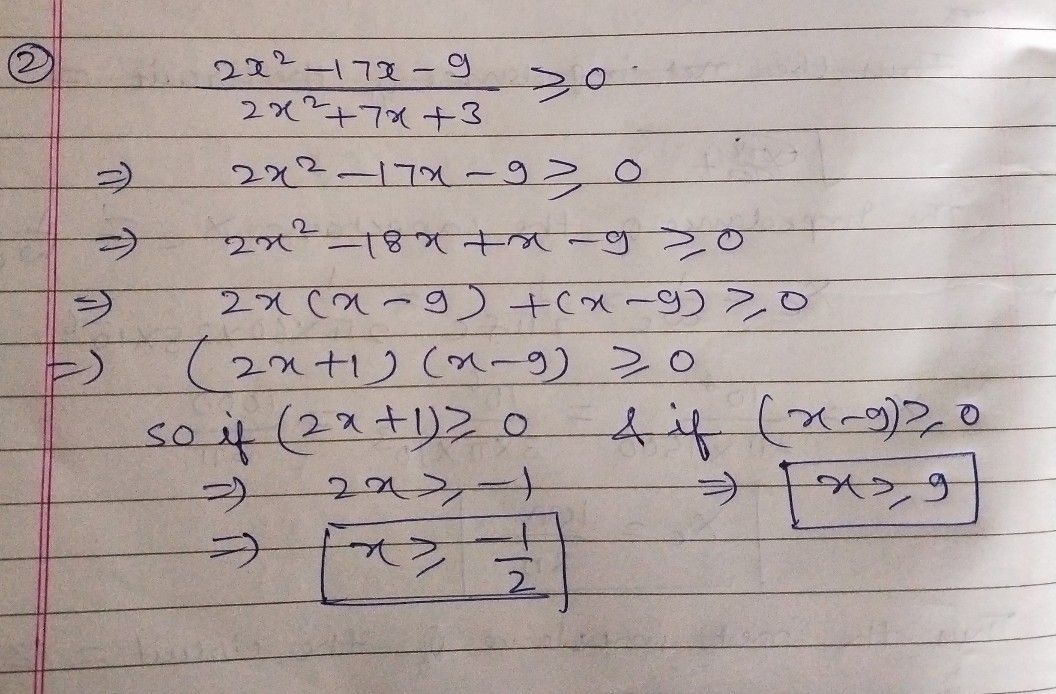Please evaluate the answer dear ?Student
oke thank uuu ma besttt teacherrrQanda teacher - SHIVRAJ
??Student
no 3&4 where teacher??Qanda teacher - SHIVRAJ
If it's helpful then give a feedback and 5star please dear ?
Ok
I am solving your problem
Please wait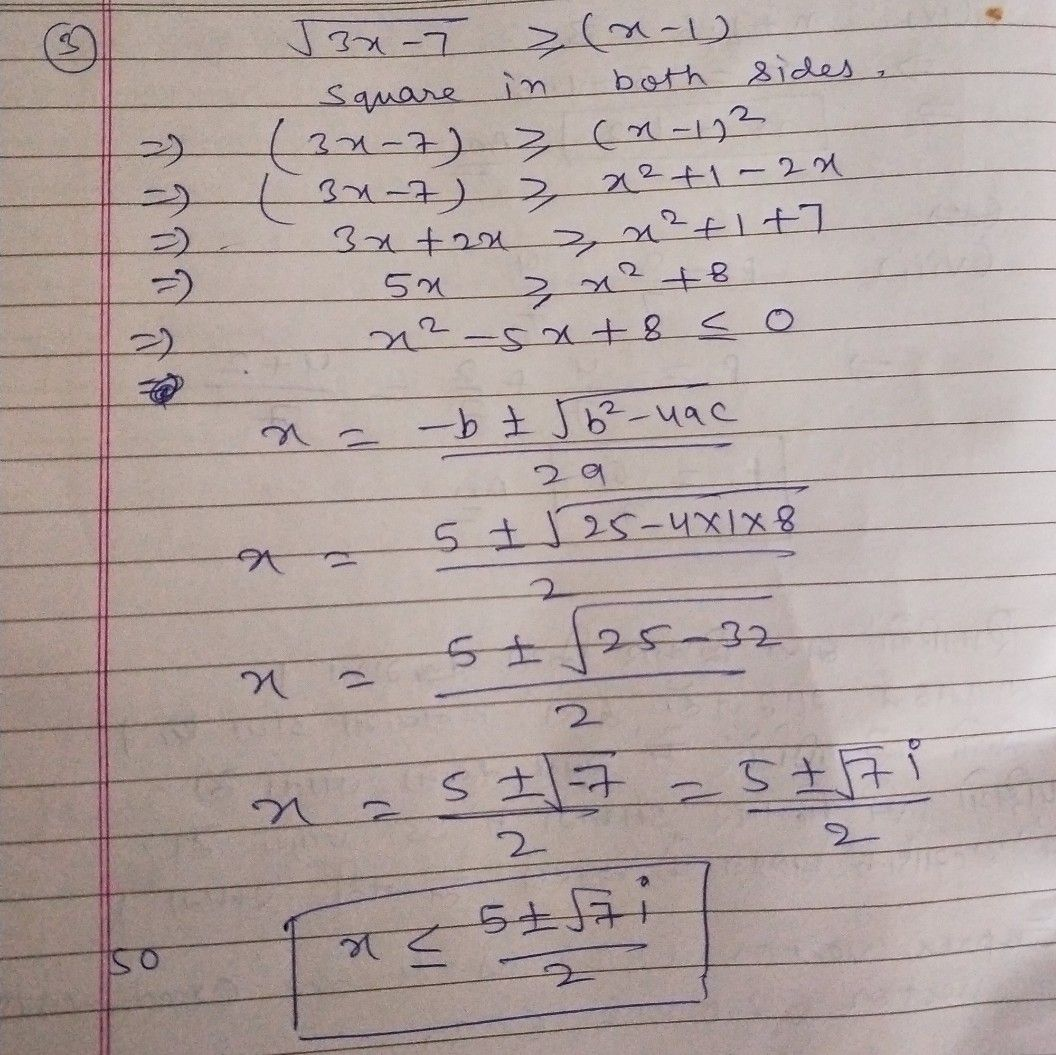Student
where to give 5 stars?Qanda teacher - SHIVRAJ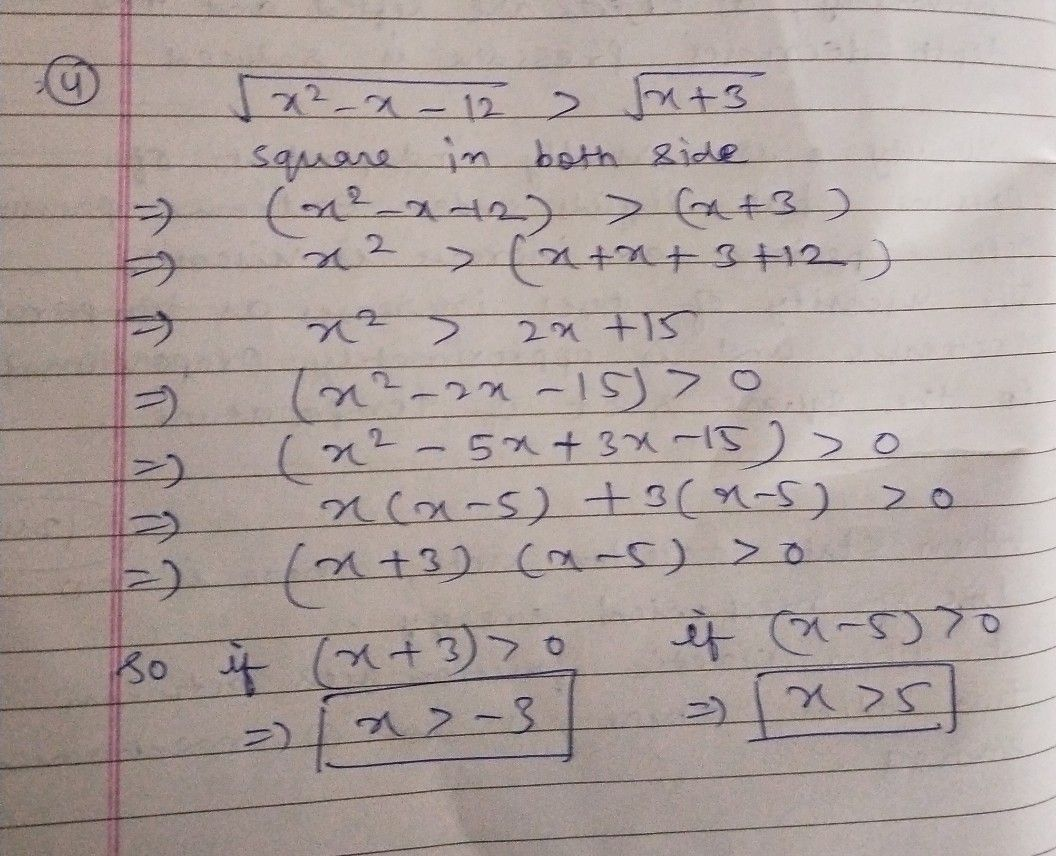If it's helpful then give a feedback and 5star please dear ?
?HereStudent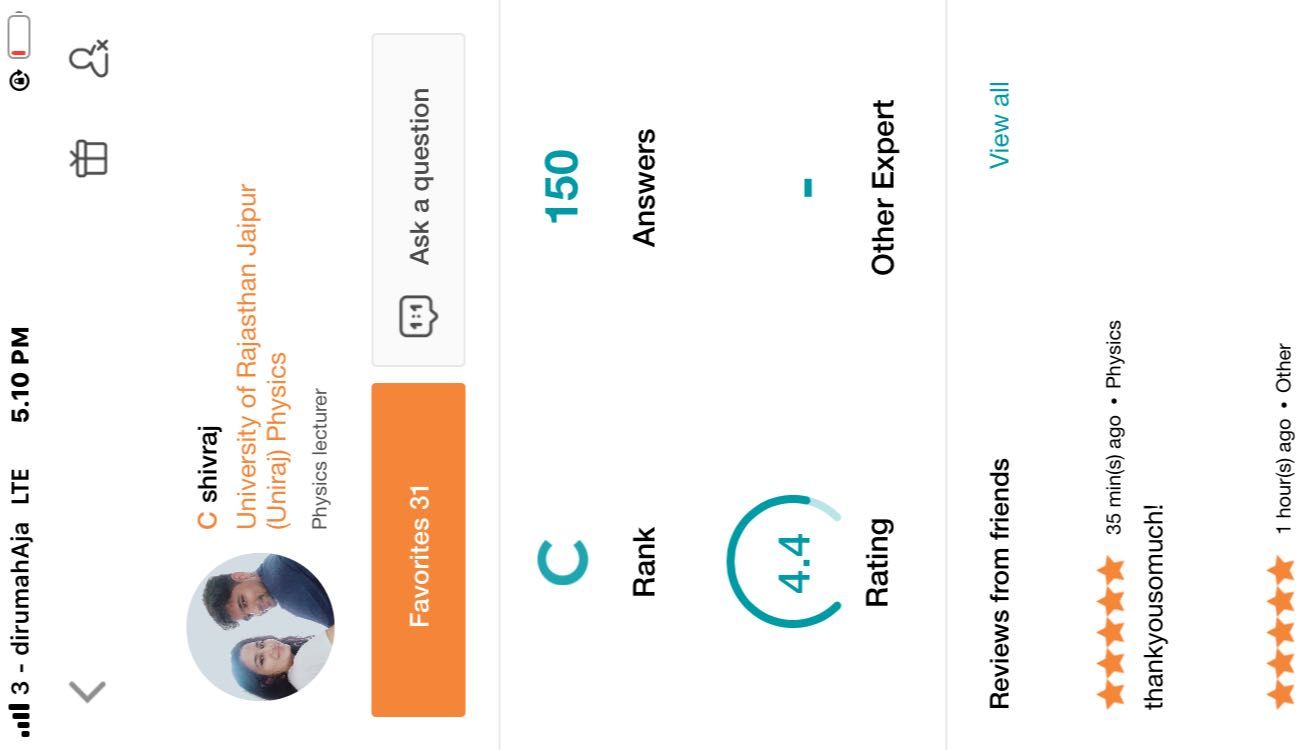where?Qanda teacher - SHIVRAJ
In chat box